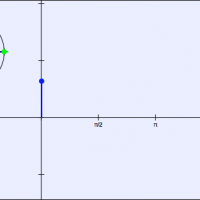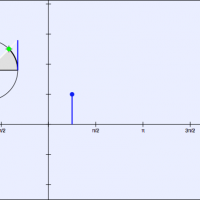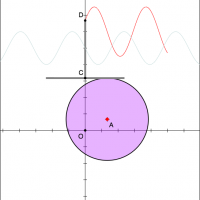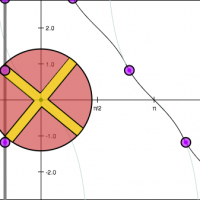## Common Core Nuggets :: Trigonometric Functions

This collection contains apps that will help teachers implement Common Core Function Standard F-TF 2, 3, 5 for High School Mathematics. Apps with an * indicate that they address the modeling standard.#### The Sine Function

This app allows the user to explore the relationship between the unit circle and the sine function. (F-TF 2, 3)#### The Cosine Function

This app allows the user to explore the relationship between the unit circle and the cosine function. (F-TF 2, 3)#### The Tangent Function

This app allows the user to explore the relationship between the unit circle and the tangent function. (F-TF 2, 3)#### Offcenter Cam*

This app explores a physical motion problem and its connection to trigonometric functions. (F-TF 5)#### Conveyor Belt*

This app explores a physical motion problem and its connection to trigonometric functions. (F-TF 5)

Apps created with Geometry Expressions